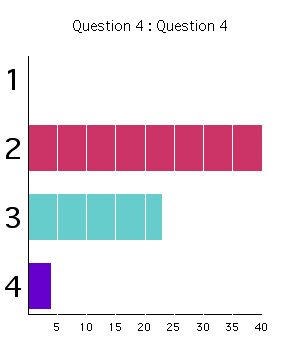Chapter 4 Question 8 Answer:

This question is designed to provide practice using the specific heat to calculate a temperature change from an energy change. It is also intended to give you some experience estimating and devloping simplified models.

For the set-up used in 2002:

The basic equation we applied was the first law with no work:

DU=Q

mcDT = Power_in * time

so time=mcDT/Power_in

When this is done for the parameters given, time = (0.3kg)(4184J/kg-K)(373K-290K)/(750J/s) = 139s. However, there were several aspects of the experiment that this does not capture. For example, energy is required to heat the beaker holding the water and the heating coils, some of the heat from the heating coils escapes below and to the sides of the beaker, and energy is carried away by the steam. Further, we can't be certain of the accuracy of the rating on the hot plate as pointed out by Prof. Hall. Therefore it is necessary to make an adjustment to our estimate to account for these effects. The more experience you have with both the physical processes and the specific test hardware, the more capable you will be to make such an adjustment. For my part I guessed that the heat capacity (mass times specific heat) of the beaker and the coils was about 30% of the water and that about 50% of the 750 watts was lost from the system either due to evaporation or heat convecting around the beaker without heating it. When the calculation is re-done with these adjustments the result is (1.3)*(0.3kg)(4184J/kg-K)(373K-290K)/(375J/s) = 361s. This is much closer to the measured result of about 355 seconds (and indeed closer than you should expect for an estimate with the very rough adjustments I made -- I just got lucky).

Class Response (2003): (Note, experimental set-up a little different than described above.)Class Response (2002):